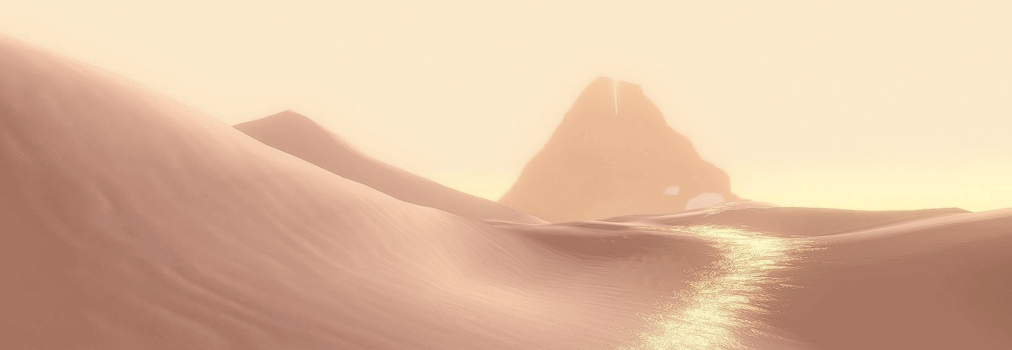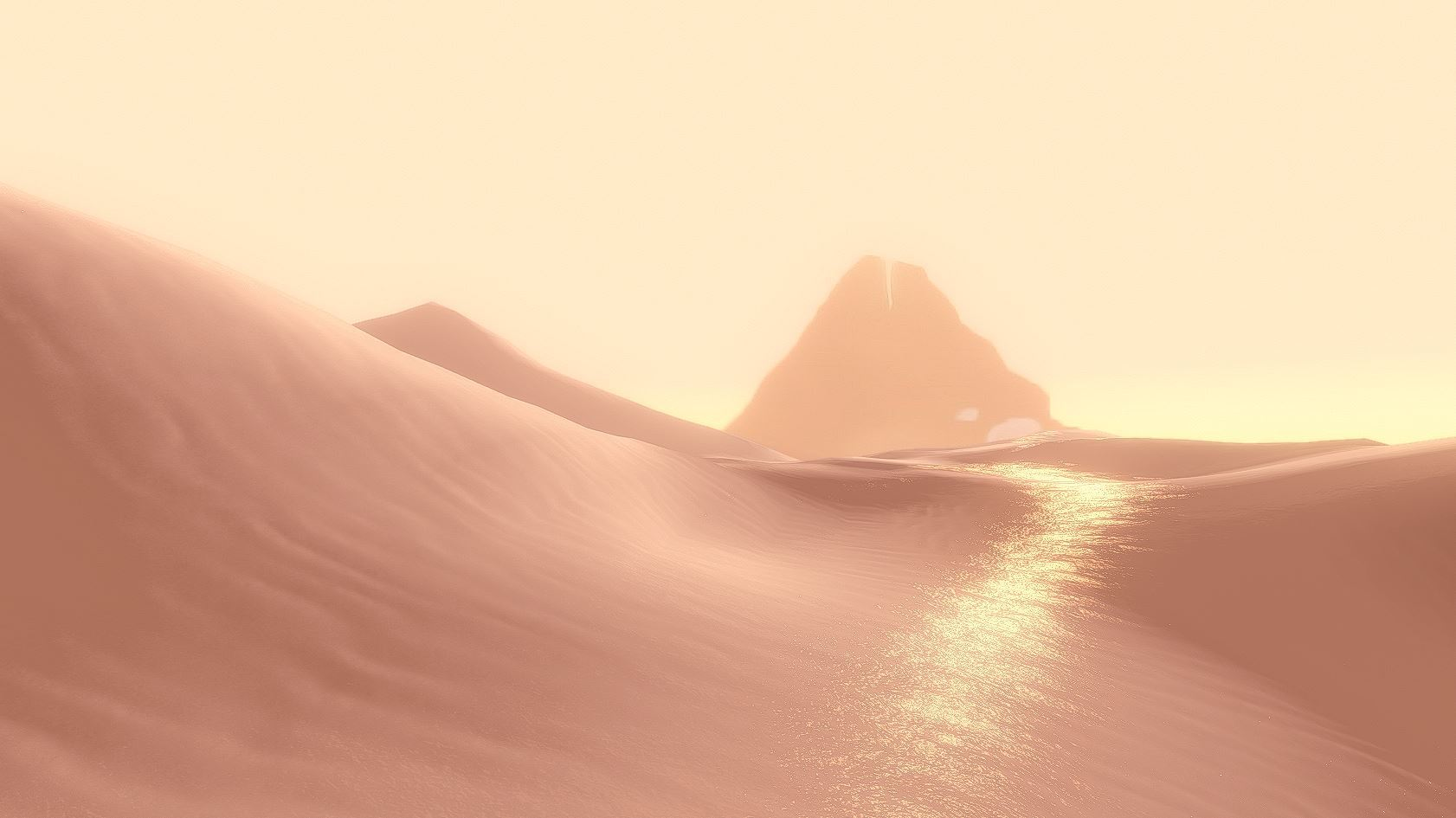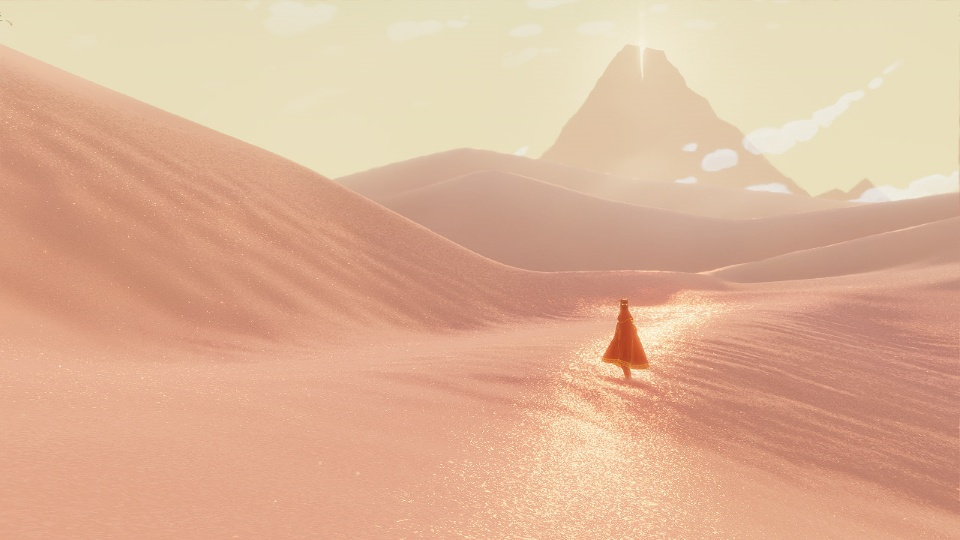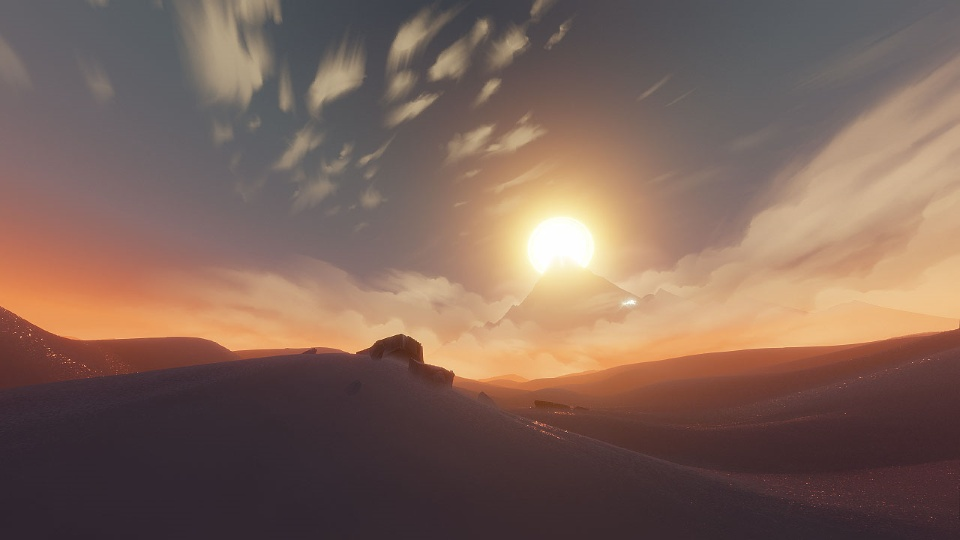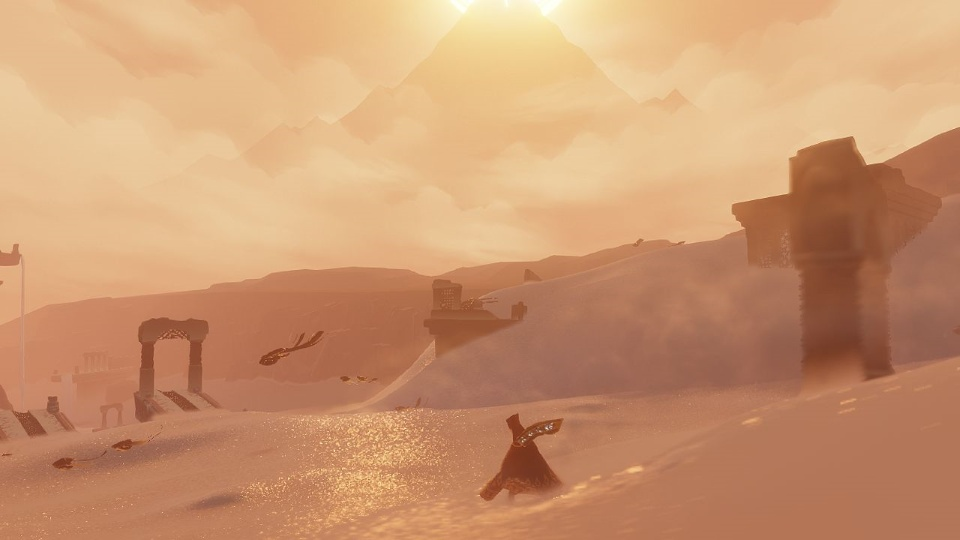## 一、建模 Modeling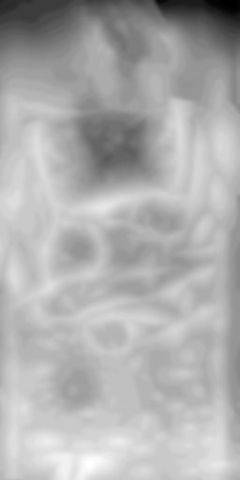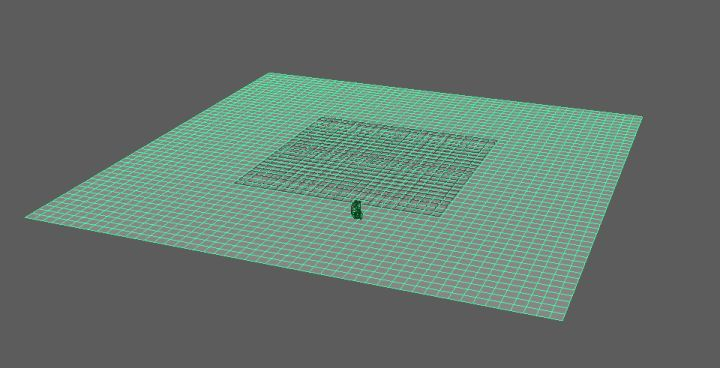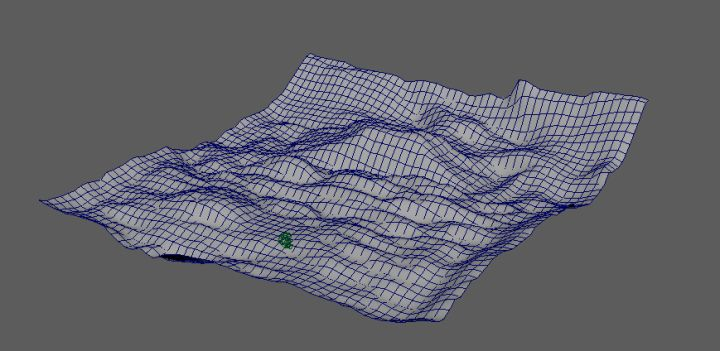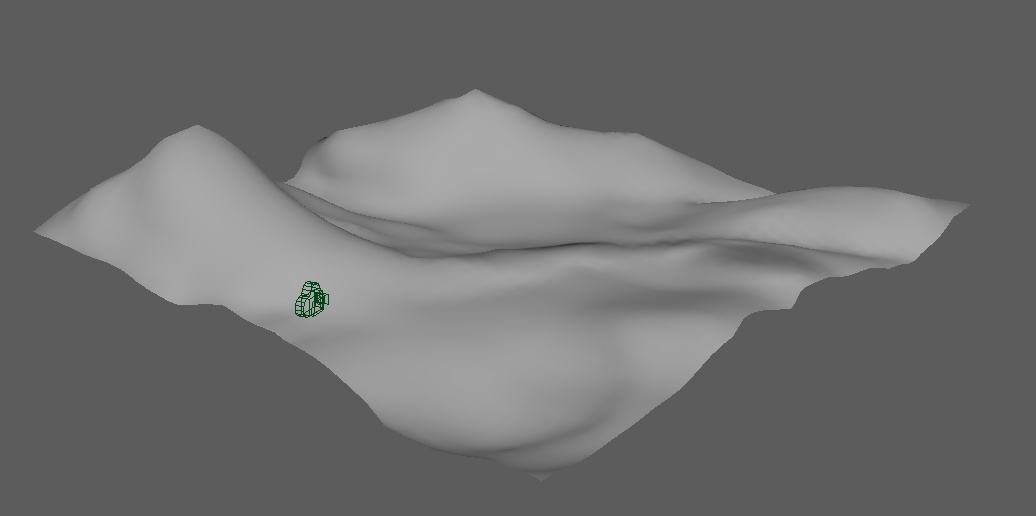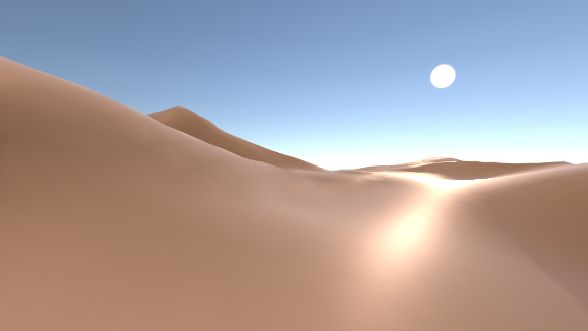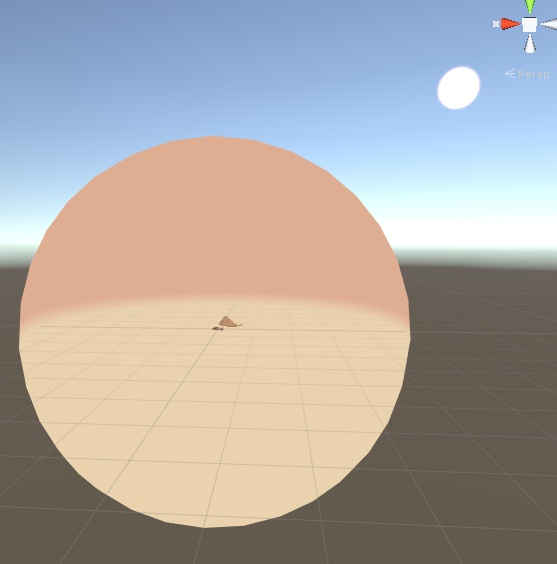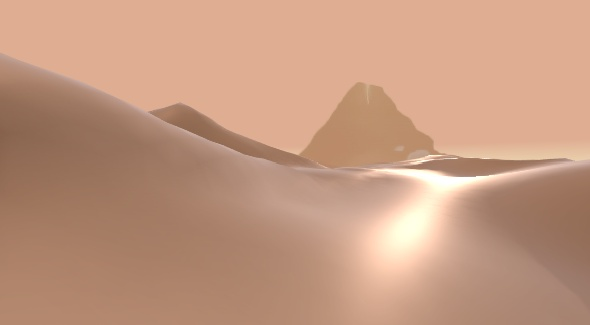## 二、基本的明暗 Diffuse

``````struct appdata
{
float4 vertex : POSITION;
float2 uv : TEXCOORD0;
float3 normal : NORMAL;
float4 tangent :TANGENT;
};

struct v2f
{
float2 uv : TEXCOORD0;
float3 worldPos : TEXCOORD1;       // position of this vertex in world
float3 view : TEXCOORD2;           // view direction, from this vertex to viewer
float3 tangentDir : TEXCOORD3;     // tangent direction in world
float3 bitangentDir : TEXCOORD4;   // bitangent direction in world

float3 normal : NORMAL;
UNITY_FOG_COORDS(5)
float4 vertex : SV_POSITION;
};
v2f vert (appdata v)
{
v2f o;
o.vertex = UnityObjectToClipPos(v.vertex);
o.uv = v.uv; // here we don't want the main texture to affect the uv
o.worldPos = mul(unity_ObjectToWorld ,v.vertex).xyz;
o.view = normalize(WorldSpaceViewDir(v.vertex));
o.normal = normalize( mul( unity_ObjectToWorld ,  v.normal).xyz ) ;
o.tangentDir = normalize( mul( unity_ObjectToWorld , float4( v.tangent.xyz, 0) ).xyz );
o.bitangentDir = normalize( cross( o.normal , o.tangentDir) * v.tangent.w );

UNITY_TRANSFER_FOG(o,o.vertex);
return o;
}
``````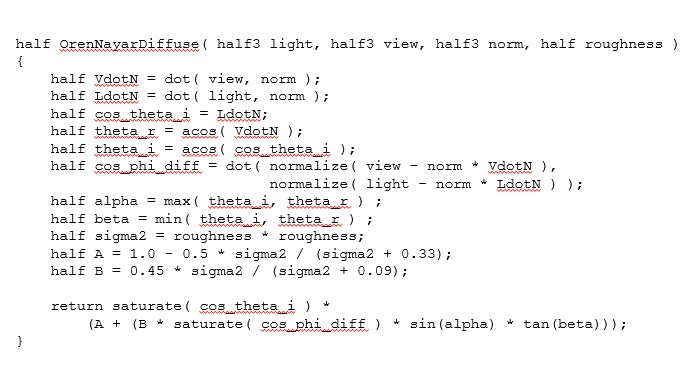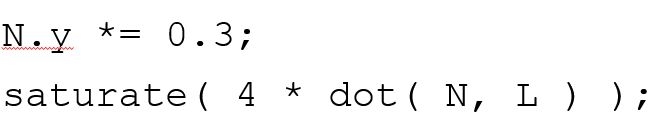``````fixed OrenNayarDiffuse( fixed3 light, fixed3 view, fixed3 norm, fixed roughness )
{
half VdotN = dot( view , norm );

// the function is modifed here
// the original one is LdotN = saturate( dot ( light , norm ))
half LdotN = saturate( 4 * dot( light, norm * float3( 1 , 0.3 , 1 ) ));
half cos_theta_i = LdotN;
half theta_r = acos( VdotN );
half theta_i = acos( cos_theta_i );
half cos_phi_diff = dot( normalize( view - norm * VdotN ),
normalize( light - norm * LdotN ) );
half alpha = max( theta_i, theta_r ) ;
half beta = min( theta_i, theta_r ) ;
half sigma2 = roughness * roughness;
half A = 1.0 - 0.5 * sigma2 / (sigma2 + 0.33);
half B = 0.45 * sigma2 / (sigma2 + 0.09);

return saturate( cos_theta_i ) *
(A + (B * saturate( cos_phi_diff ) * sin(alpha) * tan(beta)));
}
``````

``````fixed4 frag( v2f i ): SV_Target
{
float4 mainColor = tex2D( _MainTex ,  _MainTex_ST.xy * i.uv.xy + _MainTex_ST.zw );
float3 lightDirection = normalize( UnityWorldSpaceLightDir( i.worldPos ) );
float4 lightColor = _LightColor0;
float3 viewDirection = normalize( i.view );
float3 halfDirection = normalize( viewDirection + lightDirection);
float4 ambientCol = unity_AmbientSky;

float4 diffuseColor = lightColor * mainColor * OrenNayarDiffuse( lightDirection , viewDirection , normal , _Roughness) ;

return diffuseColor
}
``````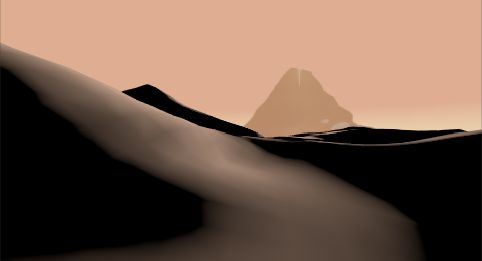``````for ( int k = 1 ; k < 4 ; ++k ) { // handle up to 4 lights
float4 lightColork = unity_LightColor[k];
float3 lightDirectionk = unity_LightPosition[k].xyz - i.worldPos * unity_LightPosition[k].w;
if ( lightColork.x + lightColork.y + lightColork.z >0 )
{
float4 diffuseColk = lightColork * mainColor * ( OrenNayarDiffuse( lightDirectionk , viewDirection ,  normal , _Roughness) );
diffuseCol += diffuseColk;
}
}
``````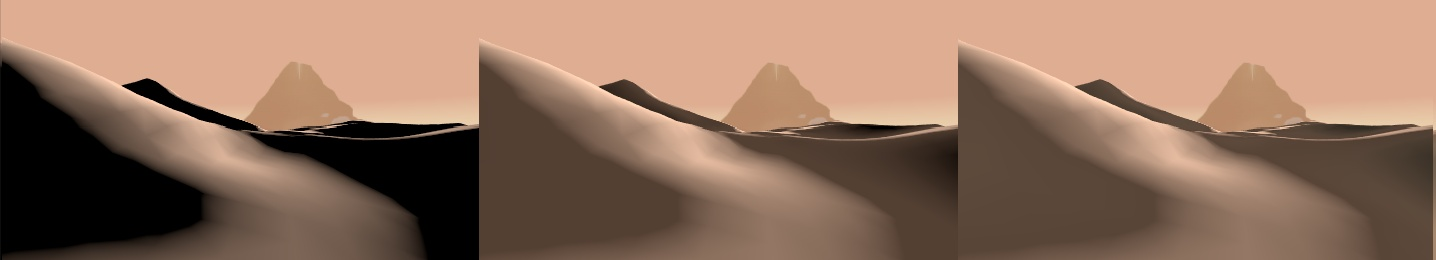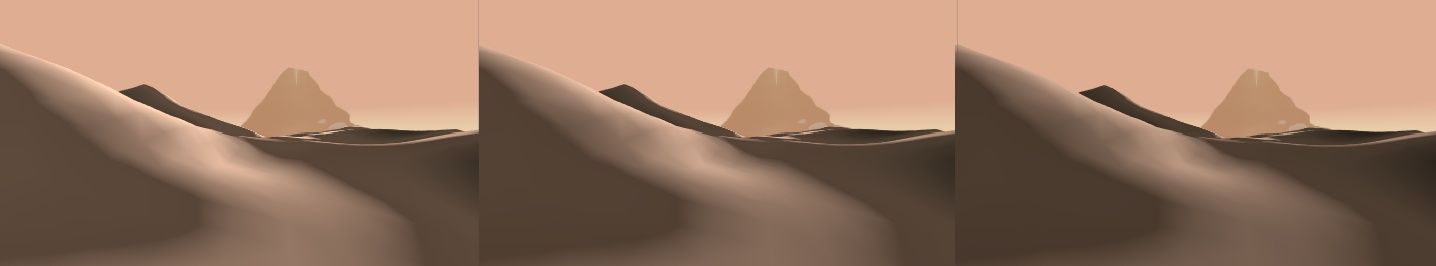## 三、沙丘表面纹理 Height Map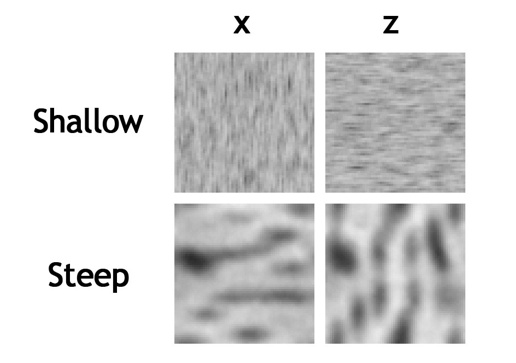X Z 方向的贴图用于不同法线朝向的表面，Steep 和 Shallow贴图分别用于不同的坡度的表面。（原话为：For each vertex of the detail heightmap, we chose the X- or the Z-column based on which derivative was greater, and we lerped between the shallow and steep rows based on the total steepness of the terrain.）

``````if ( _IsNormalXZ > 0 )
{
if ( abs( temNormal.z / temNormal.x ) > 1  )
return float3( abs( temNormal.z )  , 0 , 0  );
else
return float3( 0 , 0 , abs( temNormal.x ) );
}
``````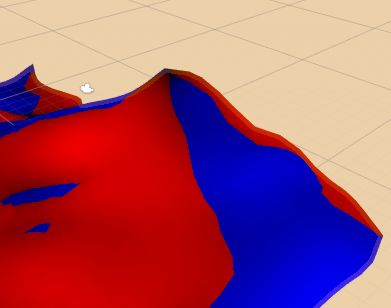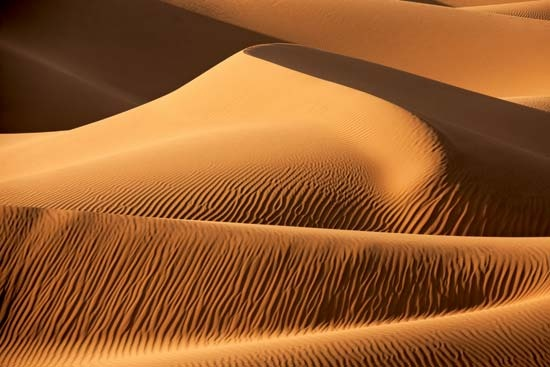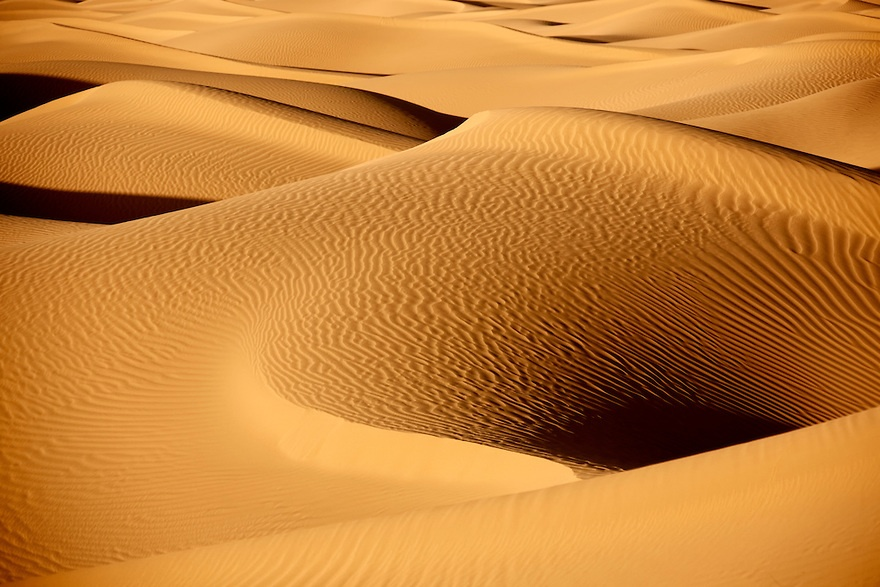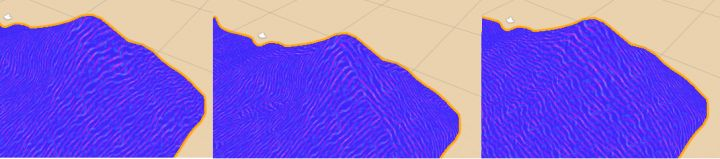``````float3 GetSurfaceNormal( float2 uv , float3 temNormal )
{
// get the power of xz direction
float xzRate = atan( abs( temNormal.z / temNormal.x) ) ;

float3 steepX = UnpackNormal( tex2D( _NormalMapSteepX , _NormalMapSteepX_ST.xy * uv.xy + _NormalMapSteepX_ST.zw ) ) ;
float3 steepZ = UnpackNormal( tex2D( _NormalMapSteepZ , _NormalMapSteepZ_ST.xy * uv.xy + _NormalMapSteepZ_ST.zw ) ) ;
return lerp( steepX , steepZ , xzRate ) ;

}
``````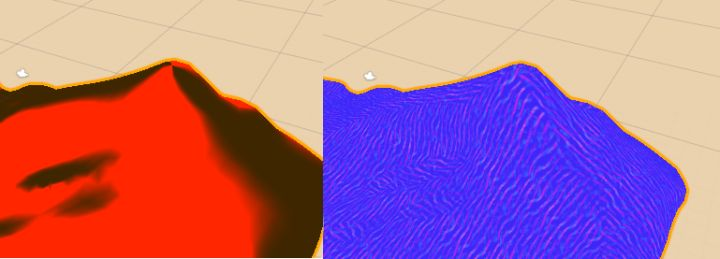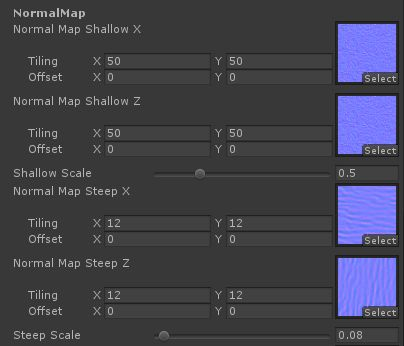``````fixed4 frag(v2f i ):SV_Target {
...

// Get the surface normal detected by the normal map
float3 normalSurface = normalize(GetSurfaceNormal( i.uv  , i.normal ) );

// 'TBN' transform the world space into a tangent space
// with the inverse matrix, we can transport the normal from tangent space to world
float3x3 TBN = float3x3( normalize( i.tangentDir ) , normalize( i.bitangentDir ) , normalize( i.normal ));
TBN = transpose( TBN);

// equals to i.tangent * ns.x + i.bitangent * ns.y + i.normal * ns.z
float3 normal = mul( TBN , normalSurface );

// Merge the surface normal with the model normal
normal = normalize( normal * _SurfaceNormalScale + i.normal);

...
}
``````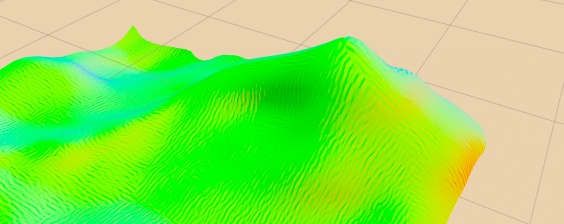``````float3 GetSurfaceNormal( float2 uv , float3 temNormal )
{
// get the power of xz direction
// it repersent the how much we should show the x or z texture
float xzRate = atan( abs( temNormal.z / temNormal.x) ) ;
xzRate = saturate( pow( xzRate , 9 ) );

// get the steepness
// the shallow and steep texture will be lerped based on this value
float steepness = atan( 1/ temNormal.y );
steepness = saturate( pow( steepness , 2 ) );

float3 shallowX = UnpackNormal( tex2D( _NormalMapShallowX , _NormalMapShallowX_ST.xy * uv.xy + _NormalMapShallowX_ST.zw ) ) ;
float3 shallowZ = UnpackNormal( tex2D( _NormalMapShallowZ , _NormalMapShallowZ_ST.xy * uv.xy + _NormalMapShallowZ_ST.zw ) ) ;
float3 shallow = shallowX * shallowZ * _ShallowBumpScale;

float3 steepX = UnpackNormal( tex2D( _NormalMapSteepX , _NormalMapSteepX_ST.xy * uv.xy + _NormalMapSteepX_ST.zw ) ) ;
float3 steepZ = UnpackNormal( tex2D( _NormalMapSteepZ , _NormalMapSteepZ_ST.xy * uv.xy + _NormalMapSteepZ_ST.zw ) ) ;
float3 steep = lerp( steepX , steepZ , xzRate ) ;

return normalize( lerp( shallow , steep , steepness ) );
}
``````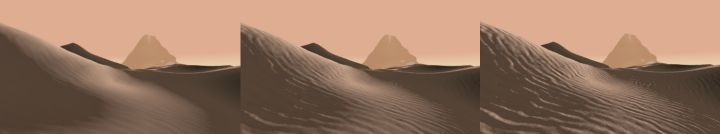## 四、风格化高光 Ocean Specualar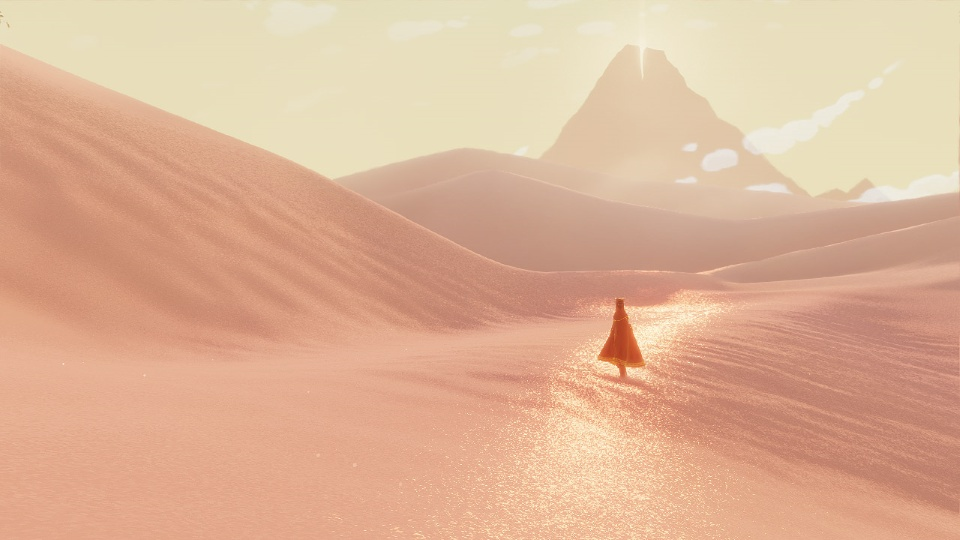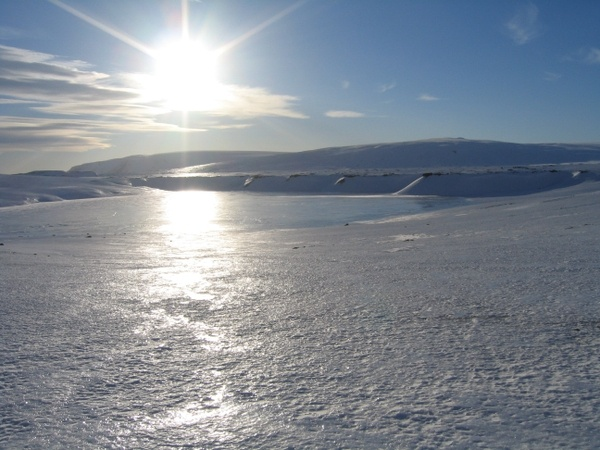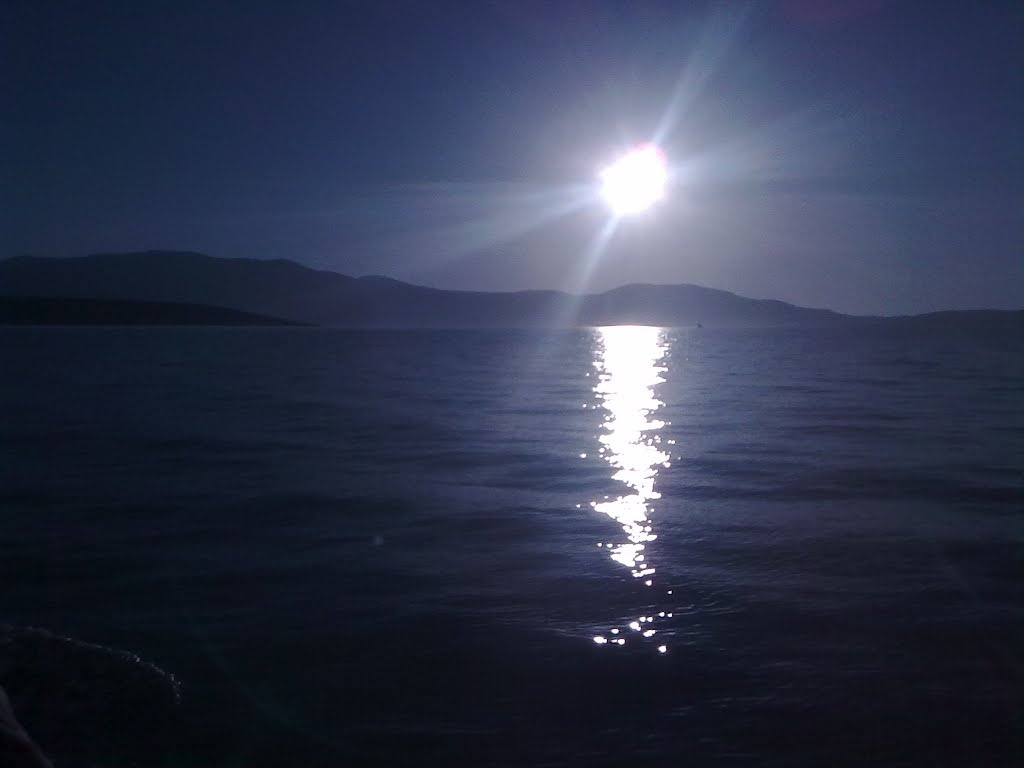``````float MySpecularDistribution( float roughness, float3 lightDir , float3 view , float3 normal , float3 normalDetail )
{
// using the blinn model
// base shine come use the normal of the object
// detail shine use the normal from the detail normal image
float3 halfDirection = normalize( view + lightDir);

float baseShine = pow(  max( 0 , dot( halfDirection , normal  ) ) , 10 / baseRoughness );
float shine = pow( max( 0 , dot( halfDirection , normalDetail  ) ) , 10 / roughness )  ;

return baseShine * shine;
}
``````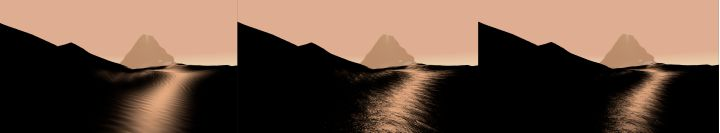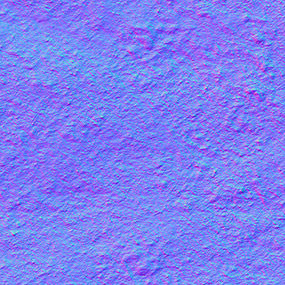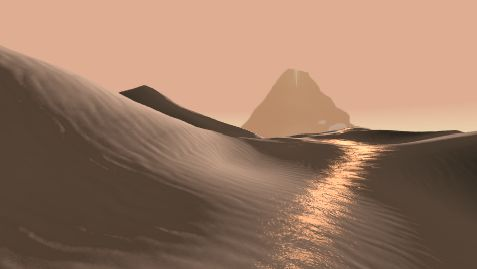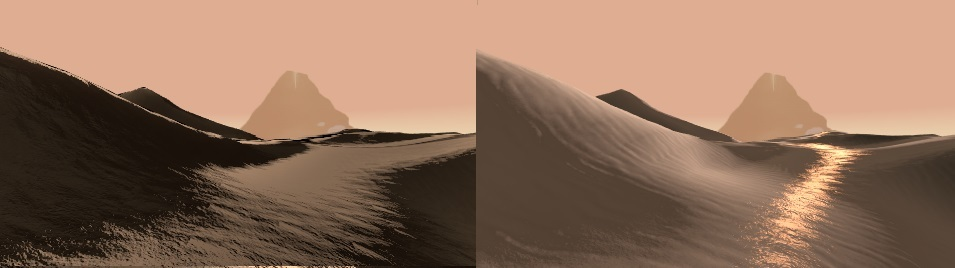## 五、贴图过滤 Anisotropic Filtering（略）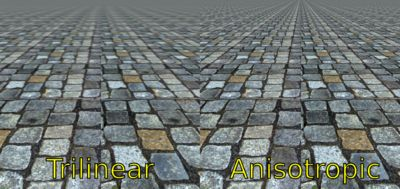## 六、亮片效果 Glitter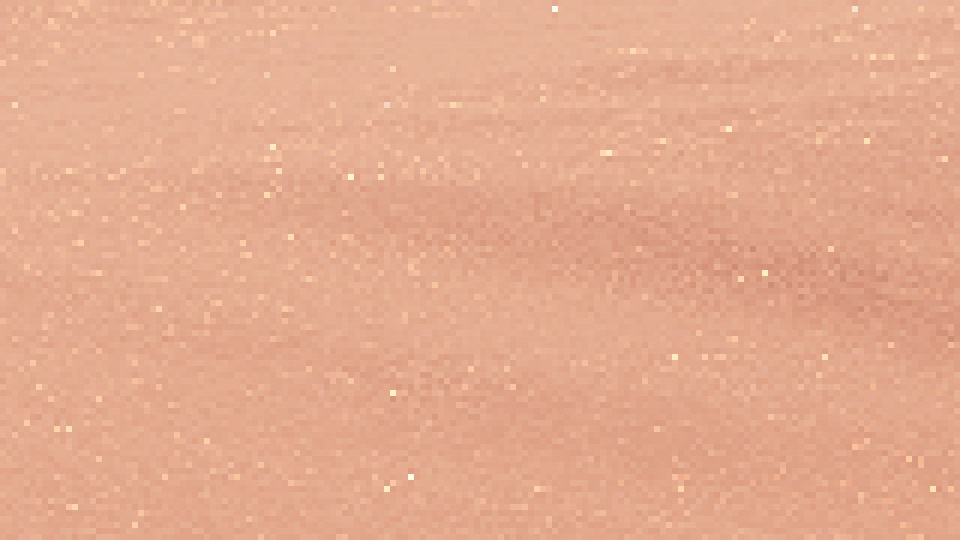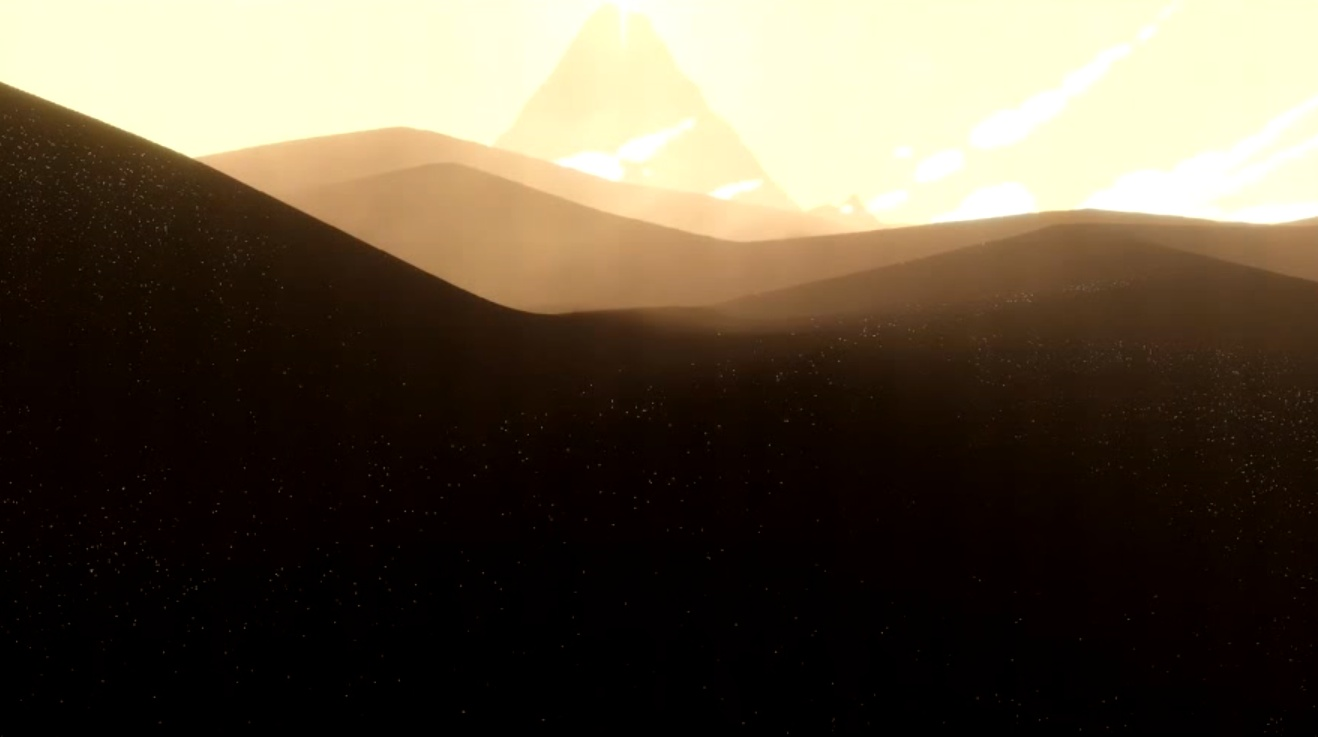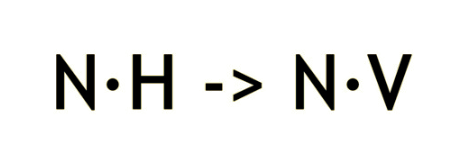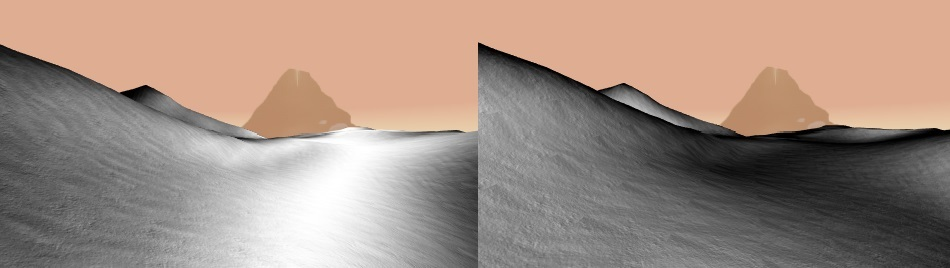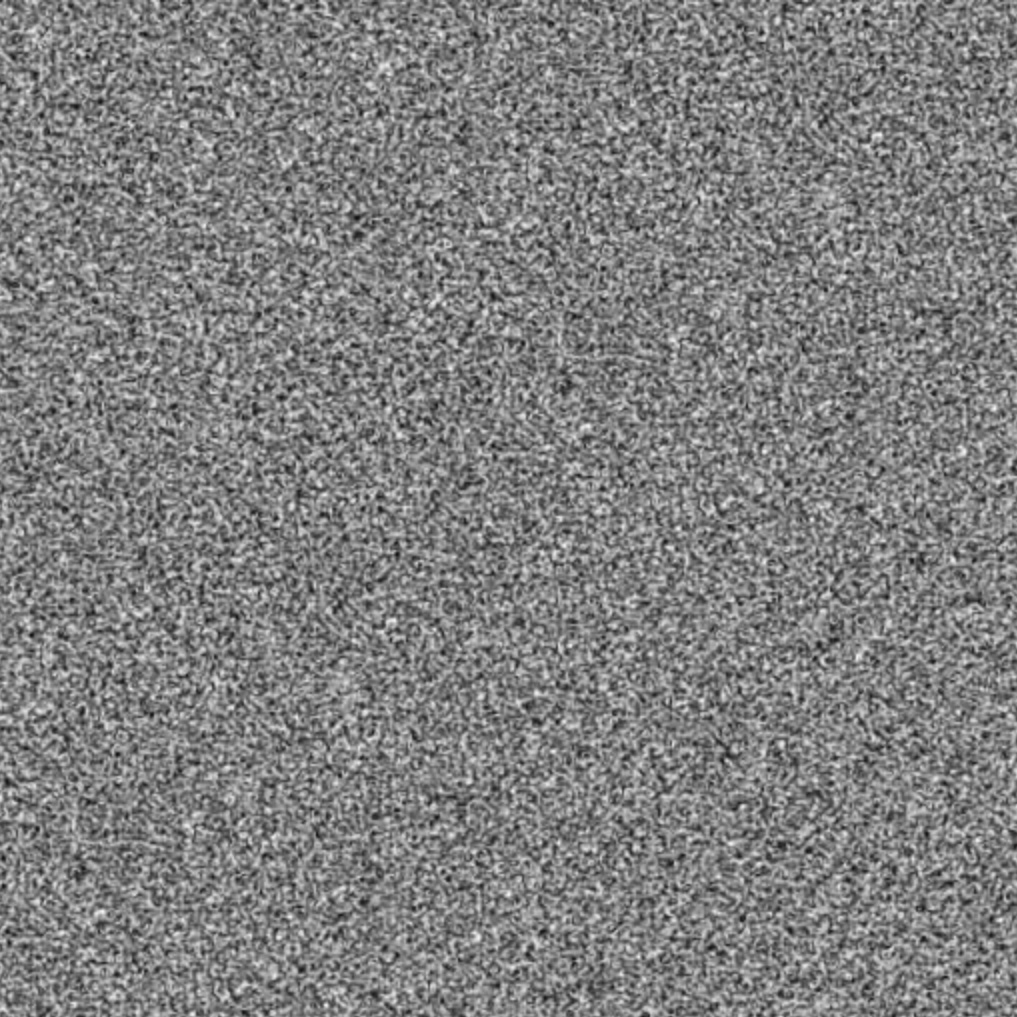``````float3 GetGlitterNoise( float2 uv )
{
return tex2D( _GlitterTex , _GlitterTex_ST.xy * uv.xy + _GlitterTex_ST.zw ) ;
}
float GliterDistribution( float Glitterness , float3 lightDir , float3 normal, float3 view , float2 uv , float3 pos )
{
...
float p1 = GetGlitterNoise( uv + float2 ( 0 , _Time.y * 0.0005 + view.x * 0.0050  )).r;
float p2 = GetGlitterNoise( uv + float2 ( _Time.y *0.001 , _Time.y * 0.001 + view.y * 0.003 )).g;

float sum = p1 * p2;
// making discrete noise
float glitter = max( 0 , pow( sum , _Glitterness ) * _GlitterMutiplyer - 0.5 ) * 2;

...
}
``````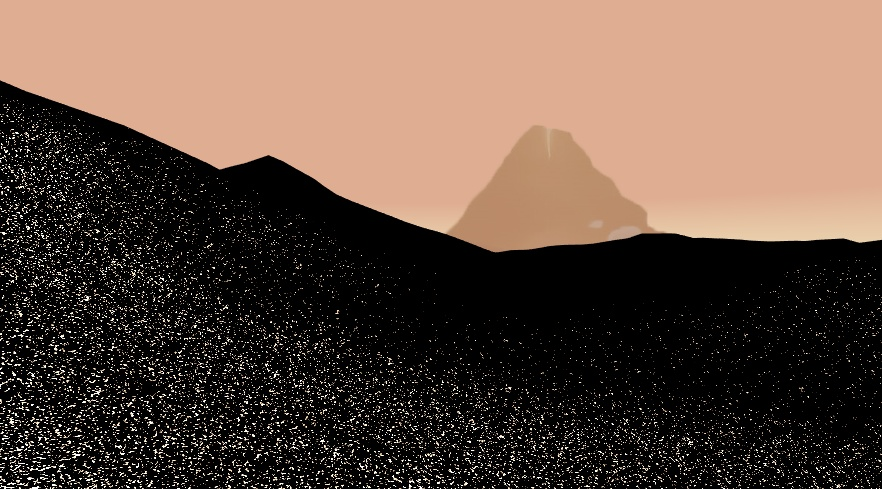``````float GliterDistribution( float3 lightDir , float3 normal, float3 view , float2 uv , float3 pos )
{
float specBase = saturate( 1 - dot( normal , view ) * 2 );
float specPow = pow( specBase , 10 / _GlitterRange );

//  A very random function to modify the glitter noise
float p1 = GetGlitterNoise( uv + float2 ( 0 , _Time.y * 0.001 + view.x * 0.006 )).r;
float p2 = GetGlitterNoise( uv + float2 ( _Time.y * 0.0006 , _Time.y * 0.0005 + view.y * 0.004  )).g;
float sum = 4 * p1 * p2;

float glitter = pow( sum , _Glitterness );
glitter = max( 0 , glitter * _GlitterMutiplyer - 0.5 ) * 2;

float sparkle = glitter * specPow;

return sparkle;
}
``````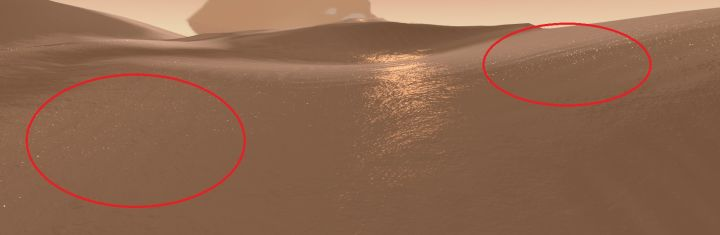## 七、其他

Mipmap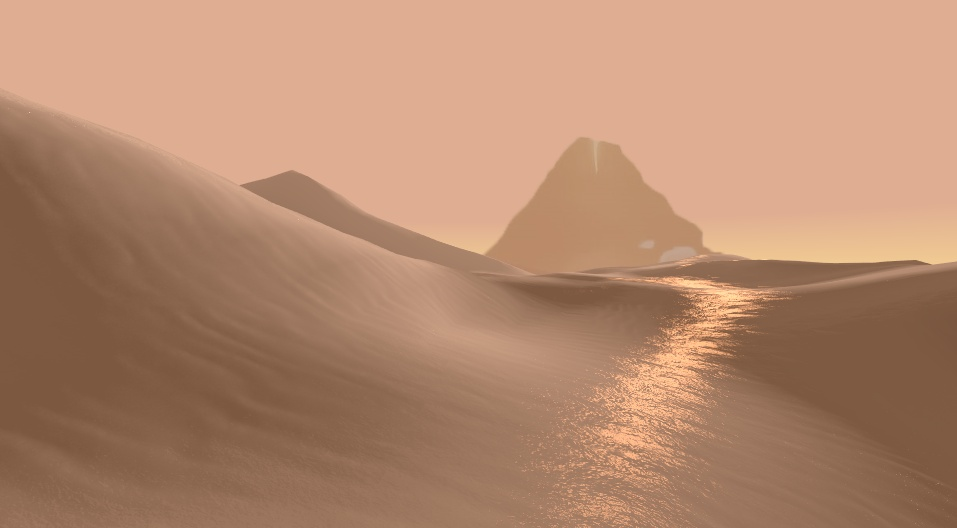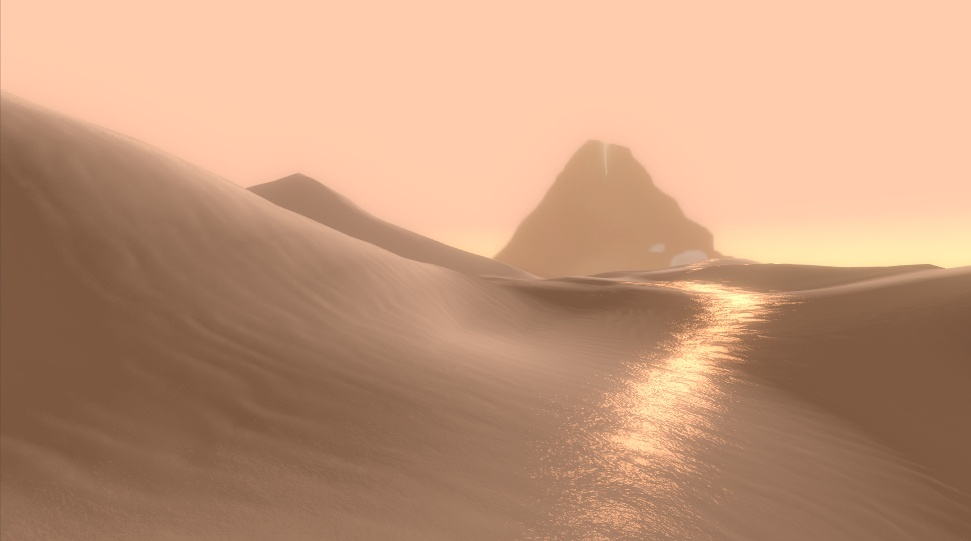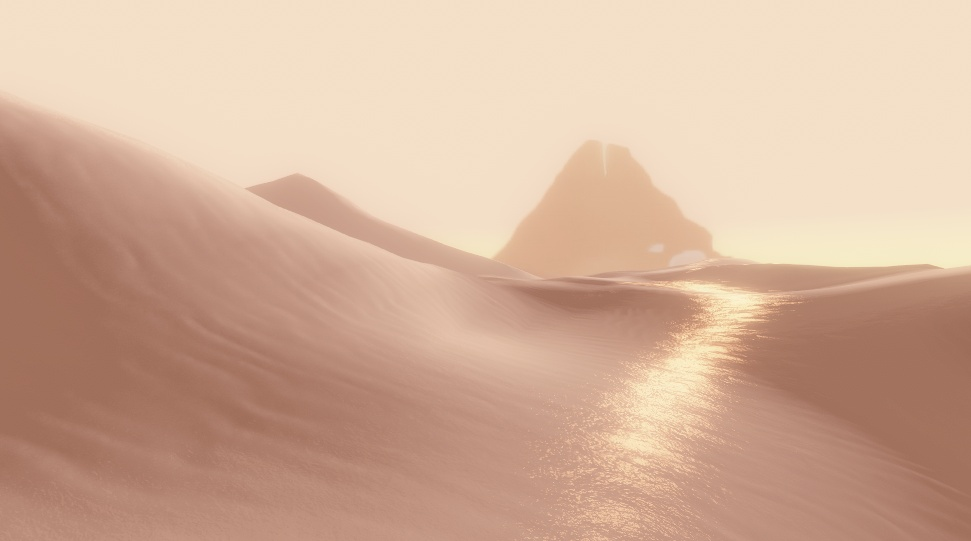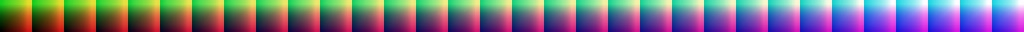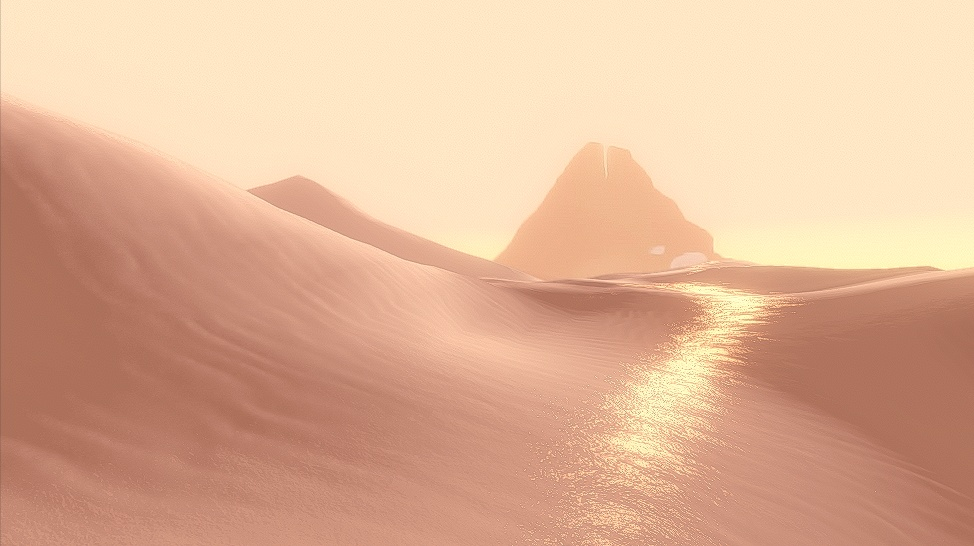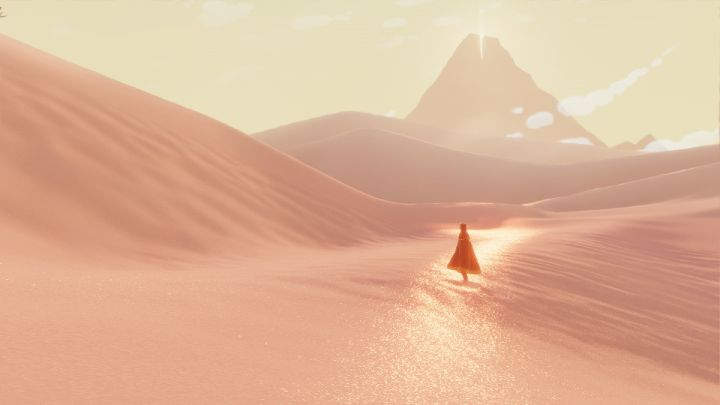https://github.com/AtwoodDeng/JourneySand